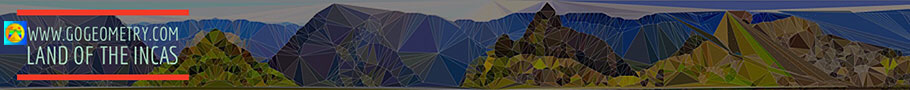# Geometry Tutoring, Tutorial, Tutor: Triangle Centers, Theorems and Problems

 Triangle Centers - Table of ContentIncenter of a triangle. Index. Excenter. Index. Excircle, Exradius. Circumcenter. Index. Centroid /Barycenter. Index. Orthocenter Index. Circumcircle Index. Nine-Point Center, Nine-Point Circle, Euler Line. Interactive illustration. Circumcenter, Centroid, Orthocenter HTML5 Animation for iPad and Nexus Adobe Flash Animation. Gergonne Points Index Triangle Center Nagel Points Index Triangle Center Gergonne Point Theorem. Concurrency. Interactive proof with animation. HTML5 Animation for Tablets (iPad, Nexus) Adobe Flash Animation Key concept: Ceva's Theorem. Nagel Point Theorem. Proof. Symmedian (Lemoine) Point Triangle Center Geometry Problem 1492. Right Triangle, Altitude, Incenters, Angle, Measurement. Geometry Problem 1491. Cyclic Quadrilateral, Diagonal, Incircle, Angle, Measurement. Geometry Problem 1490. Triangle, Cevian, Incircle, Tangent, Measurement. Geometry Problem 1489. Right Triangle, Angle Bisectors, Perpendicular, Measurement. Geometry Problem 1488. Right Triangle, Altitude, Incircle, Tangent, Measurement. Geometry Problem 1487. Right Triangle, Altitude, Incircle, Tangent, Measurement. Geometry Problem 1486. Right Triangle, Altitude, Incircle, Tangent, Measurement. Geometry Problem 1485. Triangle, Orthocenter, Altitude, Circle, Diameter, Tangent, Measurement. Dynamic Geometry 1480. Japanese Theorem for Cyclic Polygon, Sangaku, Triangulation, Non-intersecting Diagonals, Sum of Inradii, Invariant, Step-by-step Illustration. GeoGebra, iPad. Dynamic Geometry 1479. Triangle, Circumcircle, Angle Bisector, Perpendicular Bisector, Chord, Concyclic Points, Parallel Lines,, Step-by-step Illustration. GeoGebra, iPad. Dynamic Geometry 1478. Cyclocevian, Reuschle-Terquem Theorem, Concurrent Cevians, Triangle, Circumcircle, Secant line, Step-by-step Illustration. GeoGebra, iPad. Dynamic Geometry 1477. Miquel's Pentagram Theorem, Pentagon, Triangle, Circumcircles, Concyclic Points, Step-by-step Illustration. GeoGebra, iPad. Dynamic Geometry 1473. Kosnita's Theorem, Triangle, Four Circumcenters, Concurrent Line, Step-by-step Illustration. Dynamic Geometry 1471. Equilateral Triangle, Inside/Outside Point, Incenters, Tangency Points, Concurrent Lines, Step-by-step Illustration. Dynamic Geometry 1469. Triangle, Circumradius, Inradius, Midpoints, Arcs, Sum of Distances, Step-by-step Illustration. Dynamic Geometry 1468. Steiner's Theorem, Triangle, Circumradius, Inradius, Sum of Exradii, Step-by-step Illustration. Geometry Problem 1458. Triangle, Incircles, Excircle, Area, Step-by-step Illustration using GeoGebra. Geometry Problem 1457. Altitudes, Circles, Similarity, Product of the Inradii Lengths, iPad. Step-by-step illustration using GeoGebra. Geometry Problem 1456. Altitudes, Orthic Triangle, Circumcircle, Parallel lines, Similarity, Area, iPad. Step-by-step illustration using GeoGebra. Geometry Problem 1455. Nagel Point, Excircles, Incircle, Congruent Segments, iPad. Step-by-step illustration using GeoGebra. Puzzle: Exeter PointTriangle, Median, Circumcircle, Concurrent Lines, Euler Line. Dynamic Geometry: Exeter PointTriangle, Median, Circumcircle, Concurrent Lines, Euler Line, GeoGebra, HTML5 Animation for Tablets. Second Fermat Point: Static diagram. Second Fermat Point: Dynamic diagram. Triangle, Equilateral triangles, Concurrent lines, GeoGebra, HTML5 Animation for Tablets (iPad, Nexus) Morley's Triangle and Center Triangle Center Feuerbach Point Triangle Center iPad Apps: Apollonius Software. Feuerbach Point. Triangle, Incircle, Nine point circle, Tangent circles, Orthocenter, Midpoint. Interactive Geometry Software. Schiffler Point: Euler Lines Triangle Center The Bevan Point The circumcenter of the excentral triangle. Illustration with animation. Spieker Center Triangle Center Ajima-Malfatti Point 2 Triangle Center. Interactive illustration. Ajima-Malfatti Point 1 Triangle Center. Interactive illustration. Steiner Point Triangle Center Mittenpunkt Point Triangle Center Kosnita's Theorem. Circumcenters, Concurrent lines. Clawson Point. Orthic and Extangents Triangles, Concurrent lines. Clawson Point Puzzle, Geometry for Kids. 22 Piece Polygons Distances between Triangle Centers Index. Proposed Problem 246. Napoleon's Theorem I. External Equilateral triangles. Circulo de los Nueve Puntos: Spanish version. Interactive illustration. Dynamic Geometry Problem 1447. Outer Vecten Point. Step-by-step illustration using GeoGebra. Geometry Problem 1231 Triangle, Orthocenter, Incenter, Circumcenter, Angle Bisector, Center, Circle.

Go to Page: Previous | 1 | 2 | 3 | 4 | 5 | Next

 Home | Sitemap | Search | Geometry | Email | by Antonio GutierrezLast updated: Dec 30, 2020